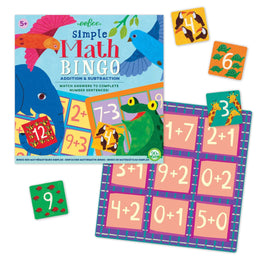••# simple maths bingo

eeBoo £14.99
• maths bingo game
• develops mathematical thinking
eeBoo simple maths bingo is a board game that introduces your child to the simple mathematical concepts of addition and subtraction. Draw a tile and reveal your number, does it answer the problem on your board? Lots of fun to be had as you race to work out sums and win the game.
+